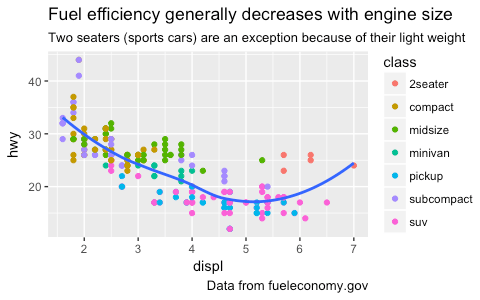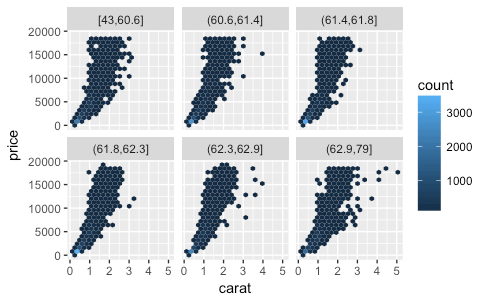# ggplot2 2.2.0 coming soon!

(This article was first published on RStudio Blog, and kindly contributed to R-bloggers)

I’m planning to release ggplot2 2.2.0 in early November. In preparation, I’d like to announce that a release candidate is now available: version 2.1.0.9001. Please try it out, and file an issue on GitHub if you discover any problems. I hope we can find and fix any major issues before the official release.

Install the pre-release version with:

``````# install.packages("devtools")

If you discover a major bug that breaks your plots, please file a minimal reprex, and then roll back to the released version with:

``install.packages("ggplot2")``

ggplot2 2.2.0 will be a relatively major release including:

• Subtitles and captions.
• A large rewrite of the facetting system.
• Improved theme options.
• Better stacking
• Numerous bug fixes and minor improvements.

The majority of this work was carried out by Thomas Pederson, who I was lucky to have as my “ggplot2 intern” this summer. Make sure to check out other visualisation packages: ggraph, ggforce, and tweenr.

## Subtitles and captions

Thanks to Bob Rudis, you can now add subtitles and captions:

``````ggplot(mpg, aes(displ, hwy)) +
geom_point(aes(color = class)) +
geom_smooth(se = FALSE, method = "loess") +
labs(
title = "Fuel efficiency generally decreases with engine size",
subtitle = "Two seaters (sports cars) are an exception because of their light weight",
caption = "Data from fueleconomy.gov"
)``````

These are controlled by the theme settings `plot.subtitle` and `plot.caption`.

The plot title is now aligned to the left by default. To return to the previous centering, use `theme(plot.title = element_text(hjust = 0.5))`.

## Facets

The facet and layout implementation has been moved to ggproto and received a large rewrite and refactoring. This will allow others to create their own facetting systems, as descrbied in the Extending ggplot2 vignette. Along with the rewrite a number of features and improvements has been added, most notably:

• Functions in facetting formulas, thanks to Dan Ruderman.
``````ggplot(diamonds, aes(carat, price)) +
geom_hex(bins = 20) +
facet_wrap(~cut_number(depth, 6))``````
• Axes were dropped when the panels in `facet_wrap()` did not completely fill the rectangle. Now, an axis is drawn underneath the hanging panels:
``````ggplot(mpg, aes(displ, hwy)) +
geom_point() +
facet_wrap(~class)``````• It is now possible to set the position of the axes through the `position` argument in the scale constructor:
``````ggplot(mpg, aes(displ, hwy)) +
geom_point() +
scale_x_continuous(position = "top") +
scale_y_continuous(position = "right")``````• You can display a secondary axis that is a one-to-one transformation of the primary axis with the `sec.axis` argument:
``````ggplot(mpg, aes(displ, hwy)) +
geom_point() +
scale_y_continuous(
"mpg (US)",
sec.axis = sec_axis(~ . * 1.20, name = "mpg (UK)")
)``````• Strips can be placed on any side, and the placement with respect to axes can be controlled with the `strip.placement` theme option.
``````ggplot(mpg, aes(displ, hwy)) +
geom_point() +
facet_wrap(~ drv, strip.position = "bottom") +
theme(
strip.placement = "outside",
strip.background = element_blank(),
strip.text = element_text(face = "bold")
) +
xlab(NULL)``````## Theming

• Blank elements can now be overridden again so you get the expected behavior when setting e.g. `axis.line.x`.
• `element_line()` gets an `arrow` argument that lets you put arrows on axes.
``````arrow <- arrow(length = unit(0.4, "cm"), type = "closed")

ggplot(mpg, aes(displ, hwy)) +
geom_point() +
theme_minimal() +
theme(
axis.line = element_line(arrow = arrow)
)``````• Control of legend styling has been improved. The whole legend area can be aligned according to the plot area and a box can be drawn around all legends:
``````ggplot(mpg, aes(displ, hwy, shape = drv, colour = fl)) +
geom_point() +
theme(
legend.justification = "top",
legend.box.margin = margin(3, 3, 3, 3, "mm"),
legend.box.background = element_rect(colour = "grey50")
)``````• `panel.margin` and `legend.margin` have been renamed to `panel.spacing` and `legend.spacing` respectively as this better indicates their roles. A new `legend.margin` has been actually controls the margin around each legend.
• When computing the height of titles ggplot2, now inclues the height of the descenders (i.e. the bits `g` and `y` that hang underneath). This makes improves the margins around titles, particularly the y axis label. I have also very slightly increased the inner margins of axis titles, and removed the outer margins.
• The default themes has been tweaked by Jean-Olivier Irisson making them better match `theme_grey()`.
• Lastly, the `theme()` function now has named arguments so autocomplete and documentation suggestions are vastly improved.

## Stacking bars

`position_stack()` and `position_fill()` now stack values in the reverse order of the grouping, which makes the default stack order match the legend.

``````avg_price <- diamonds %>%
group_by(cut, color) %>%
summarise(price = mean(price)) %>%
ungroup() %>%
mutate(price_rel = price - mean(price))

ggplot(avg_price) +
geom_col(aes(x = cut, y = price, fill = color))``````(Note also the new `geom_col()` which is short-hand for `geom_bar(stat = "identity")`, contributed by Bob Rudis.)

Additionally, you can now stack negative values:

``````ggplot(avg_price) +
geom_col(aes(x = cut, y = price_rel, fill = color))``````The overall ordering cannot necessarily be matched in the presence of negative values, but the ordering on either side of the x-axis will match.

If you want to stack in the opposite order, try `forcats::fct_rev()`:

``````ggplot(avg_price) +
geom_col(aes(x = cut, y = price, fill = fct_rev(color)))``````